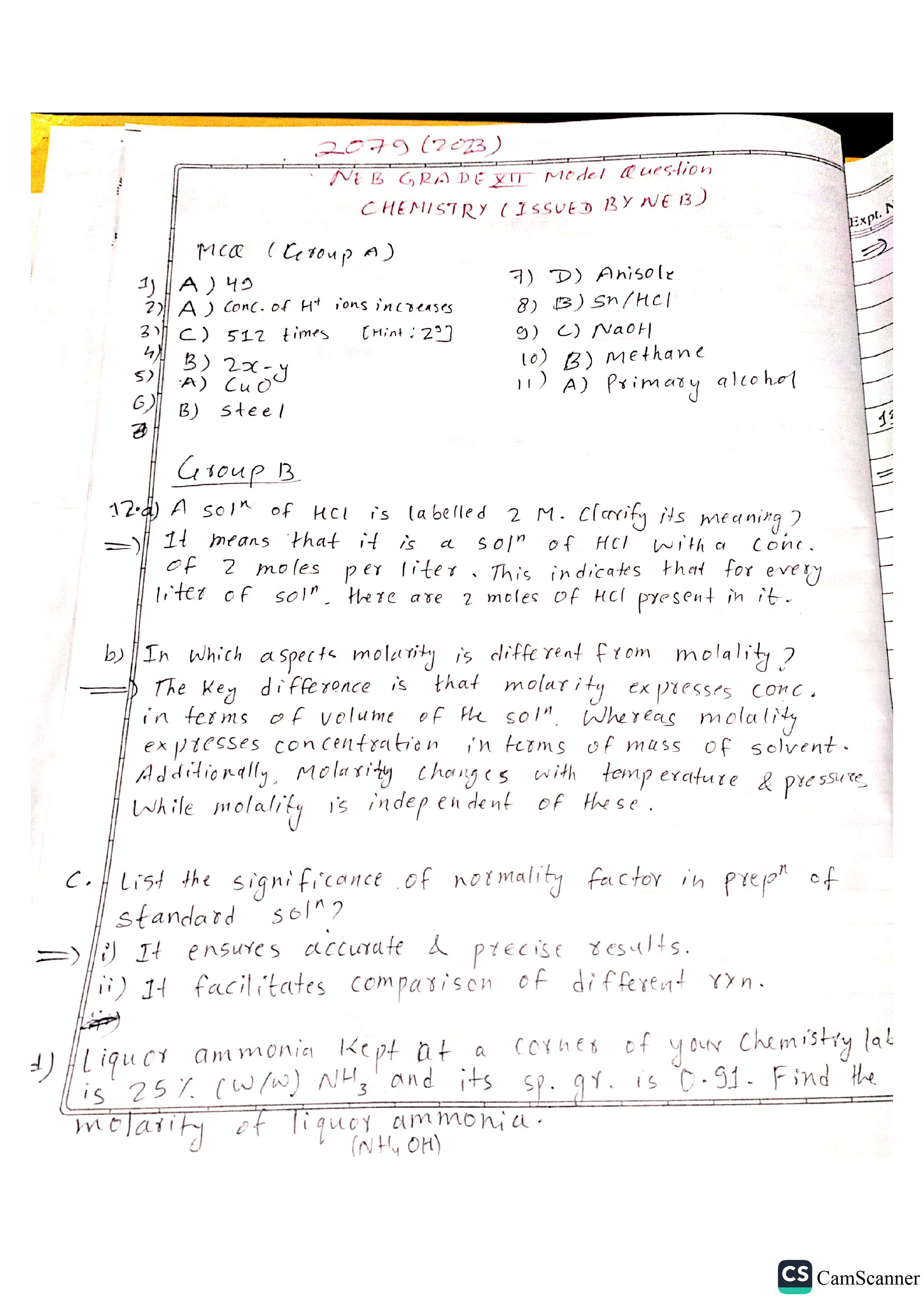# NEB Class 12 Chemistry Model Question 2080 Solution

Complete Model Question and Answer Solution of Class 12 Chemistry Model Question 2079/2080 SET Issued By NEB.

In this Post, We Provide Complete Model Question and Answer Solution of Class 12 Chemistry Model Question 2079/2080 SET Issued By NEB.

## Class 12 NEB Chemistry Model Question

Class 12 Chemistry Model Question 2079 Released by NEB for 2080 Batch Student is given as:

## Class 12 NEB Chemistry Model Question Solution 2079

Complete Answer Solution of Class 12 Chemistry Model Question Solution 2079/2080, Question by National Education Board.

MCQ

1. Identify the equivalent weight of K2Cr2O7 in the following reaction?
(Cr =52, K=39)
K2Cr2O7 + 3H2SO4 + 5(COOH) 2 K2SO4 + Cr2 {SO4)3 + 10CO2 + 8H2O

• A) 49 B) 294
• C) 98 D) 108

2. In a solution that is at equilibrium, what happens to the concentration of H+ ions if the concentration of OH- ions is increased?

• A) The concentration of H+ ions increases
• B) The concentration of H+ ions decreases
• C) The concentration of H+ ions stay the same
• D) It depends on the initial concentration of H+ ions

Answer: B) The concentration of H+ ions decreases

3. Assuming the rate of a reaction is doubled for every 10oC rise in temperature how many times increases the rate of temperature rises from 100C to 1000C?

A) 112 times B) 400 times C) 512 times D) 614 times

4. for the given reactions
i. C + O2→ CO2, ∆ H = - x KJmol-1
ii. 2CO + O2→ 2CO2, ∆ H = - y KJmol-1
The enthalpy of formation of CO becomes

A) 2y-x  B) 2x-y C) (y−2x)/2 D) (x−2y)/2

5. What product would be obtained if red hot copper wire reacts with steam?

A)CuO B) Cu2O C) Cu2O2 D) Cuo2

6. For which manufacturing process, Bessemer converter is used?

A) Pig iron B) Steel C) Wrought iron D) Cast iron

7. When Sodium phenoxide reacts with methyl bromide it gives

A) Cresol B) Toluene C) benzene D) Anisole

8. Identify the X in the following reaction:

A) Na2S B) Sn/HCl C) LiAlH4 D) Na/H2

9. Which of the following reagents can be used to distinguish between a phenol and a carboxylic acid?

A) KOH B) Na C) NaOH D) NaHCO3

10. The colorless sweet smelling liquid compound A which exposed in air forms poisonous phosgene and also react with acetone gives sleep-inducing drug. Predict the product when the compound A reduced in a neutral medium?

A) Methylene chloride B) Methane C) Ethyne D) Ethane

11. Oxygen containing organic compounds upon oxidation forms a carboxylic acid as the major organic product with its molecular mass higher by 14 units. Identify the organic compound.

A) A primary alcohol B) An aldehyde C) A ketone. D) A secondary alcoholYou Can download Complete Pdf Solution by Abhi Chaudhary [fb], of Class 12 Chemistry Model Question Solution by clicking on download now below.

Getting Info...

Iswori Rimal is the author of iswori.com.np, a popular education platform in Nepal. Iswori helps students in their SEE, Class 11 and Class 12 studies with Complete Notes, important questions and other study materials.

1. I think the correct option of MCQ no 2 should be
Option B) The concentration of H+ ions decreases
1. yes yes , thankyou;
In a solution at equilibrium, if the concentration of OH- ions is increased, the concentration of H+ ions will decrease. This is because in an equilibrium reaction between an acid and a base, an increase in the concentration of one of the products will cause a decrease in the concentration of the other product, in order to maintain the equilibrium constant. In the case of the autoionization of water, where H2O molecules can react with each other to form H+ and OH- ions, an increase in OH- concentration will cause a decrease in H+ concentration to maintain the equilibrium constant for the reaction:

2H2O ⇌ H3O+ + OH-

Therefore, the correct answer is B) The concentration of H+ ions decreases.
2. In qsn no 8 a
when nitrobenzene reacts with LiAlH4 the product will be azobenzene not aniline!
1. The correct answer is B) Sn/HCl.

To convert 1,3-dinitrobenzene to 3-nitroaniline, we need to reduce the nitro groups (-NO2) to amino groups (-NH2). This can be achieved using the Sn/HCl reducing agent, which is also known as the Clemmensen reduction.

In this reaction, the nitro groups are first reduced to nitroso groups (-NO) using tin and hydrochloric acid (Sn/HCl). The nitroso groups are then further reduced to amino groups using the same reagents. The final product is 3-nitroaniline.

The other options listed (A) Na2S, (C) LiAlH4, and (D) Na/H2, are not suitable for this transformation. Na2S is typically used for thiolation reactions, LiAlH4 is a strong reducing agent that can reduce nitro groups to amines but it is not selective for 3-nitro group and it can lead to over-reduction, and Na/H2 is typically used for hydrogenation reactions.
3. Nice